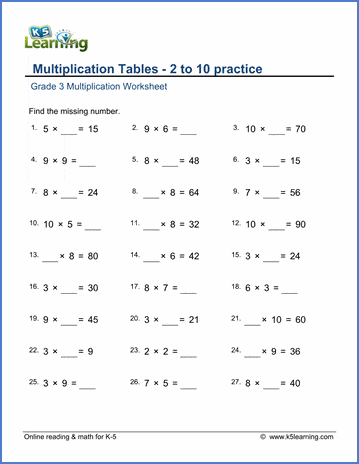# Multiplication tables (2-10) with missing factors

## Math fact worksheets: Multiplication tables of 2 to 10 with missing numbers

Below are six versions of our grade 3 math worksheet on the multiplication tables of 2 to 10 with missing factors.  Students must fill in the missing number in the multiplication equations.  These worksheets are pdf files.## More multiplication worksheets

Find all of our multiplication worksheets, from basic multiplication facts to multiplying multi-digit whole numbers in columns.

## What is K5?

K5 Learning offers reading and math worksheets, workbooks and an online reading and math program for kids in kindergarten to grade 5.  We help your children build good study habits and excel in school.